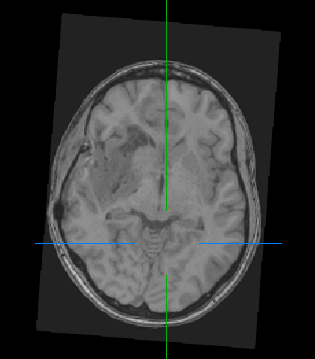# Output MRI images have value 0 (almost) everywhere after resampling using SimpleITK

Hey!

I have 3-dimensional NIfTI MRI images that I would like to resample to uniform voxel size (1, 1, 1). This works fine:

``````img = sitk.ReadImage(img_path)

output_spacing = [1.0, 1.0, 1.0]

input_spacing = img.GetSpacing()
input_size = img.GetSize()
size_x = int(round(input_size * input_spacing/output_spacing))
size_y = int(round(input_size * input_spacing/output_spacing))
size_z = int(round(input_size * input_spacing/output_spacing))
size = [size_x, size_y, size_z]

resampler = sitk.ResampleImageFilter()

resampler.SetDefaultPixelValue(0)
resampler.SetOutputDirection(img.GetDirection())
resampler.SetInterpolator(sitk.sitkLinear)
resampler.SetOutputOrigin(img.GetOrigin())
resampler.SetOutputSpacing([1.0, 1.0, 1.0])
resampler.SetSize(size)

img_resampled = resampler.Execute(img)
``````

However, the original images also look rotated:I suspect this is because they have strange directions, e.g. `(-0.08117500577885353, -0.01032194044222358, 0. 9966465738475954, 0.9617029292569339, 0.26183942332790555, 0.08103908870110368, 0.26179781186143564, -0.9650562542861514, 0.011325764448564252)`.

But if I set `resampler.SetOutputDirection([1.0, 0.0, 0.0, 1.0, 0.0, 1.0])` instead of `resampler.SetOutputOrigin(img.GetOrigin()`), the output MRT images have (almost) everywhere the value 0.

What is the reason and how can I fix this error?

Thanks in advance for any help!

Hello @bluefire,

Please see this Jupyer notebook, the `make_isotropic` function, You might find the toolkit’s notebooks repository of interest too.

Thanks for your help! I will try it!